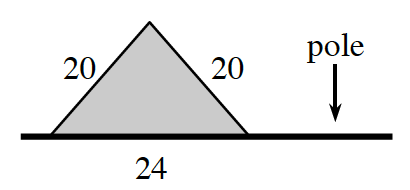### Home > APCALC > Chapter 1 > Lesson 1.4.4 > Problem1-188

1-188.The shaded region at right represents a triangular “flag.” To help you visualize this, use the ).

1. Imagine rotating this flag about its “pole” and describe the resulting three-dimensional figure. Draw a picture of this figure on your paper.

2. Calculate the volume of the rotated flag.

$\text{Volume of a Cone }=\frac{1}{3}\pi r^2h$

Use the Pythagorean Theorem (or special right triangles) to find the radius of the cone.

Use the eTool below to visualize the flag.
Click the link at right for the full version of the eTool: Calc 1-188 HW eTool